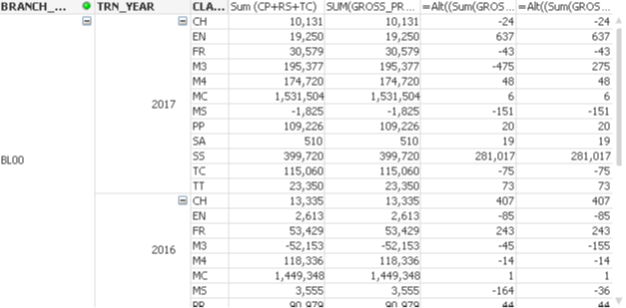QlikView App Development

Discussion Board for collaboration related to QlikView App Development.

Announcements
Valued Contributor

fabs applied seems not working!

Dear Sunny, Once growth rate was calculated normally it shows in 6th column. Since if there was a negative value last Year with positive figure in the current Year, I brought in "fabs" to overcome it but the answer seems to be wrong. Please check the answer of Class M3 , It actually needs to be 475 (positive) but takes 275.  Other figures are ok. Expression applied are too summerized  below.

/Below(TOTAL Sum( GROSS_PREMIUM), Count(DISTINCT TOTAL <TRN_YEAR>CLASS_CODE))*100, 0)

=Alt((Sum(GROSS_PREMIUM)- fabs( Below(TOTAL Sum(GROSS_PREMIUM), Count(DISTINCT TOTAL <TRN_YEAR> CLASS_CODE))))

/fabs( Below(TOTAL Sum( GROSS_PREMIUM), Count(DISTINCT TOTAL <TRN_YEAR>CLASS_CODE)))*100, 0)

Neville3 RepliesMVP

Re: fabs applied seems not working!

fabs() is probably working fine.

Could you please share your definition of a percentage change when the values to compare are of a potential different sign?

IMO, it will be very hard to define a consistent, unambigous definition.

Would be interested in seeing one.

Valued Contributor

Re: fabs applied seems not working!

Dear Stefan,

I have given the formula applied & results just check the class M3, may be the place I have added fabs not correct. If you could refer my 2nd expression with fabs word, you may see here I have gone wrong!

Regards

NevilleMVP

Re: fabs applied seems not working!

Hi Neville, maybe without the first fabs:

=Alt((Sum(GROSS_PREMIUM)- Below(TOTAL Sum(GROSS_PREMIUM), Count(DISTINCT TOTAL <TRN_YEAR> CLASS_CODE)))

/fabs( Below(TOTAL Sum( GROSS_PREMIUM), Count(DISTINCT TOTAL <TRN_YEAR>CLASS_CODE)))*100, 0)

It will compare the total difference with past year value always positive, if you do the firts fabs you are not comparing the total difference between years.